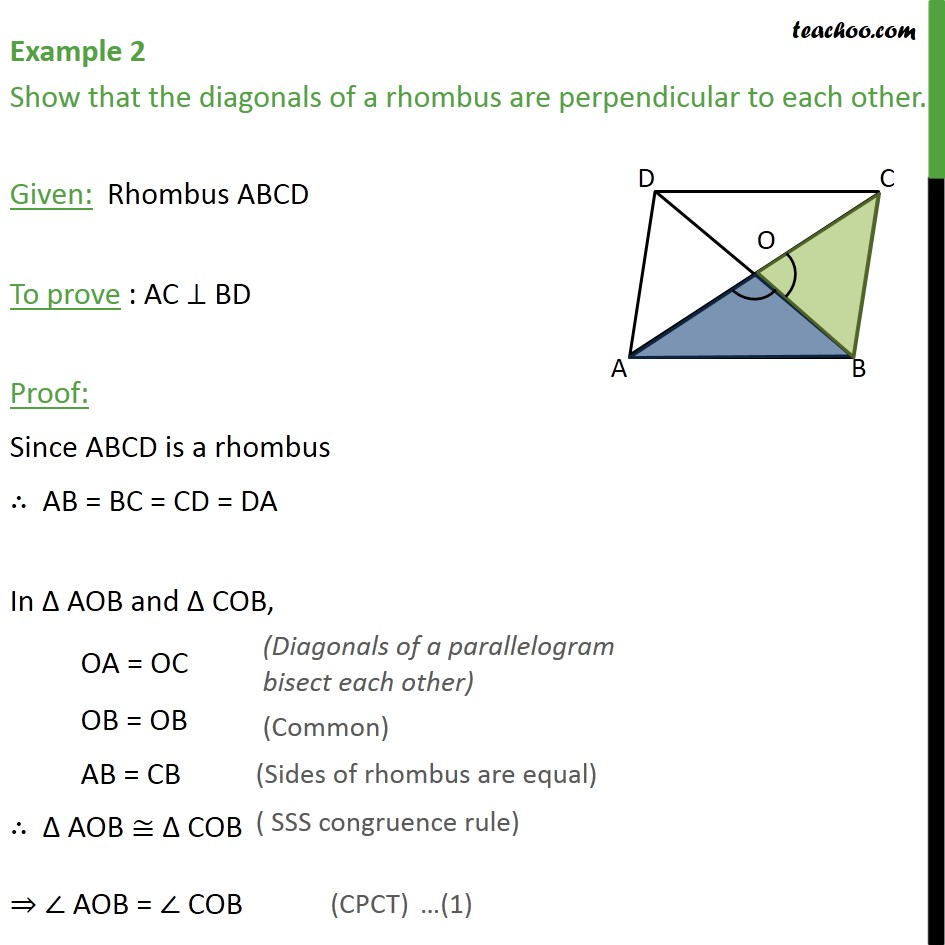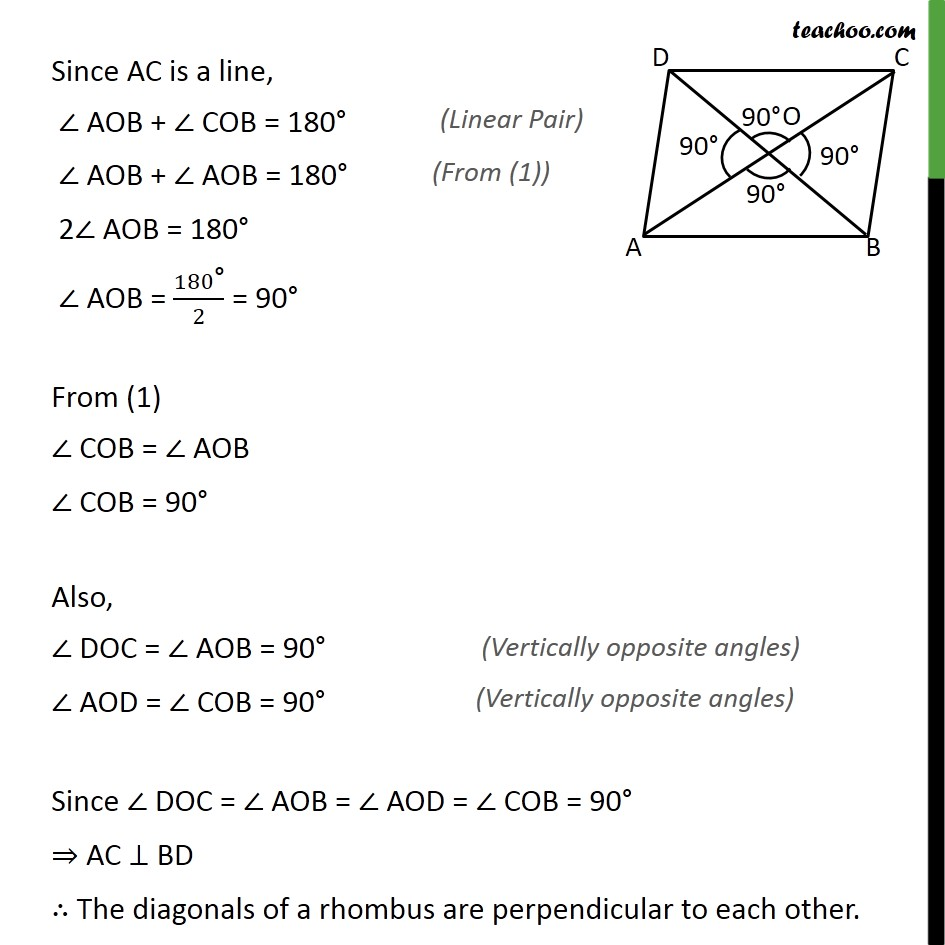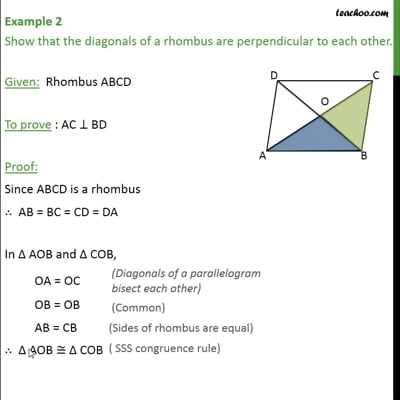Examples

Serial order wiseThis video is only available for Teachoo black users

Maths Crash Course - Live lectures + all videos + Real time Doubt solving!

### Transcript

Example 2 Show that the diagonals of a rhombus are perpendicular to each other. Given: Rhombus ABCD To prove : AC BD Proof: Since ABCD is a rhombus AB = BC = CD = DA In AOB and COB, OA = OC OB = OB AB = CB AOB COB AOB = COB Since AC is a line, AOB + COB = 180 AOB + AOB = 180 2 AOB = 180 AOB = 180" " /2 = 90 From (1) COB = AOB COB = 90 Also, DOC = AOB = 90 AOD = COB = 90 Since DOC = AOB = AOD = COB = 90 AC BD The diagonals of a rhombus are perpendicular to each other.